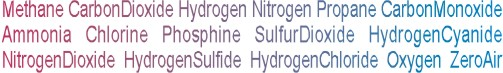Calibration gas blends, mixes and accessories
NON-REACTIVE SINGLE GAS > BUTANE :    12 Items found.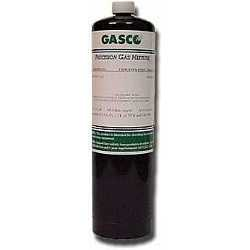(1025)

NORLAB Equivalent  P101160

Norlab Equivalent Calibration Gas 60% LEL Butane Balance air 17L Liter cylinder (Made by Gasco)

Configure

\$36.47(1024)

NORLAB Equivalent  P101150

Norlab Equivalent Calibration Gas 50% LEL Butane Balance air 17L Liter cylinder (Made by Gasco)

Configure

\$36.47(1023)

NORLAB Equivalent  P101125

Norlab Equivalent Calibration Gas 25% LEL Butane Balance air 17L Liter cylinder (Made by Gasco)

Configure

\$36.47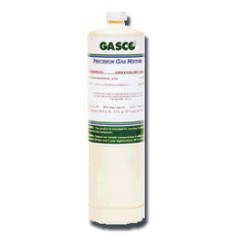(1028)

NORLAB Equivalent  HP101160

Norlab Equivalent Calibration Gas 60% LEL Butane Balance air 34LS Liter cylinder (Made by Gasco)

Configure

\$51.53(1027)

NORLAB Equivalent  HP101150

Norlab Equivalent Calibration Gas 50% LEL Butane Balance air 34LS Liter cylinder (Made by Gasco)

Configure

\$51.53(1026)

NORLAB Equivalent  HP101125

Norlab Equivalent Calibration Gas 25% LEL Butane Balance air 34LS Liter cylinder (Made by Gasco)

Configure

\$51.53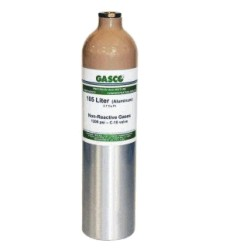(1031)

NORLAB Equivalent  101160

Norlab Equivalent Calibration Gas 60% LEL Butane Balance air 105L Liter cylinder (Made by Gasco)

Configure

\$109.41(1030)

NORLAB Equivalent  101150

Norlab Equivalent Calibration Gas 50% LEL Butane Balance air 105L Liter cylinder (Made by Gasco)

Configure

\$109.41(1029)

NORLAB Equivalent  101125

Norlab Equivalent Calibration Gas 25% LEL Butane Balance air 105L Liter cylinder (Made by Gasco)

Configure

\$109.41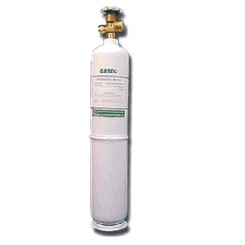(1034)

NORLAB Equivalent  E101160

Norlab Equivalent Calibration Gas 60% LEL Butane Balance air 552L Liter cylinder (Made by Gasco)

\$224.41(1033)

NORLAB Equivalent  E101150

Norlab Equivalent Calibration Gas 50% LEL Butane Balance air 552L Liter cylinder (Made by Gasco)

\$224.41(1032)

NORLAB Equivalent  E101125

Norlab Equivalent Calibration Gas 25% LEL Butane Balance air 552L Liter cylinder (Made by Gasco)

\$224.41